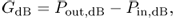Chapter 21

PA (Power Amplifier)

# 21.1 Classification of PA

A PA (power amplifier) is an amplifier that intensifies the power of an input signal (Fig. 21.1).

Figure 21.1 Representation of a power amplifier.Its output power is G times its input power. The added portion of the output power is AC power converted from the DC power supply. The ratio of the added portion of the power to the input power is called its PAE (power added efficiency), that is,

21.121.221.321.4where

Pout, watt = the output power in watts,

Pin, watt = the input power in watts,

Pout, dB = the output power in decibels,

Pin, dB = the input power in decibels,

Gwatt = the power gain in watts,

GdB = the power gain in decibels,

ΔP = the difference between output and input power in watts,

PDC = the DC power from the DC power supply, and

PAE = the power added efficiency in percent.

In a PA design, the most important goal is the power conversion efficiency from DC power to AC power, which combined with the input power ...

Get RF Circuit Design, 2nd Edition now with the O’Reilly learning platform.

O’Reilly members experience books, live events, courses curated by job role, and more from O’Reilly and nearly 200 top publishers.